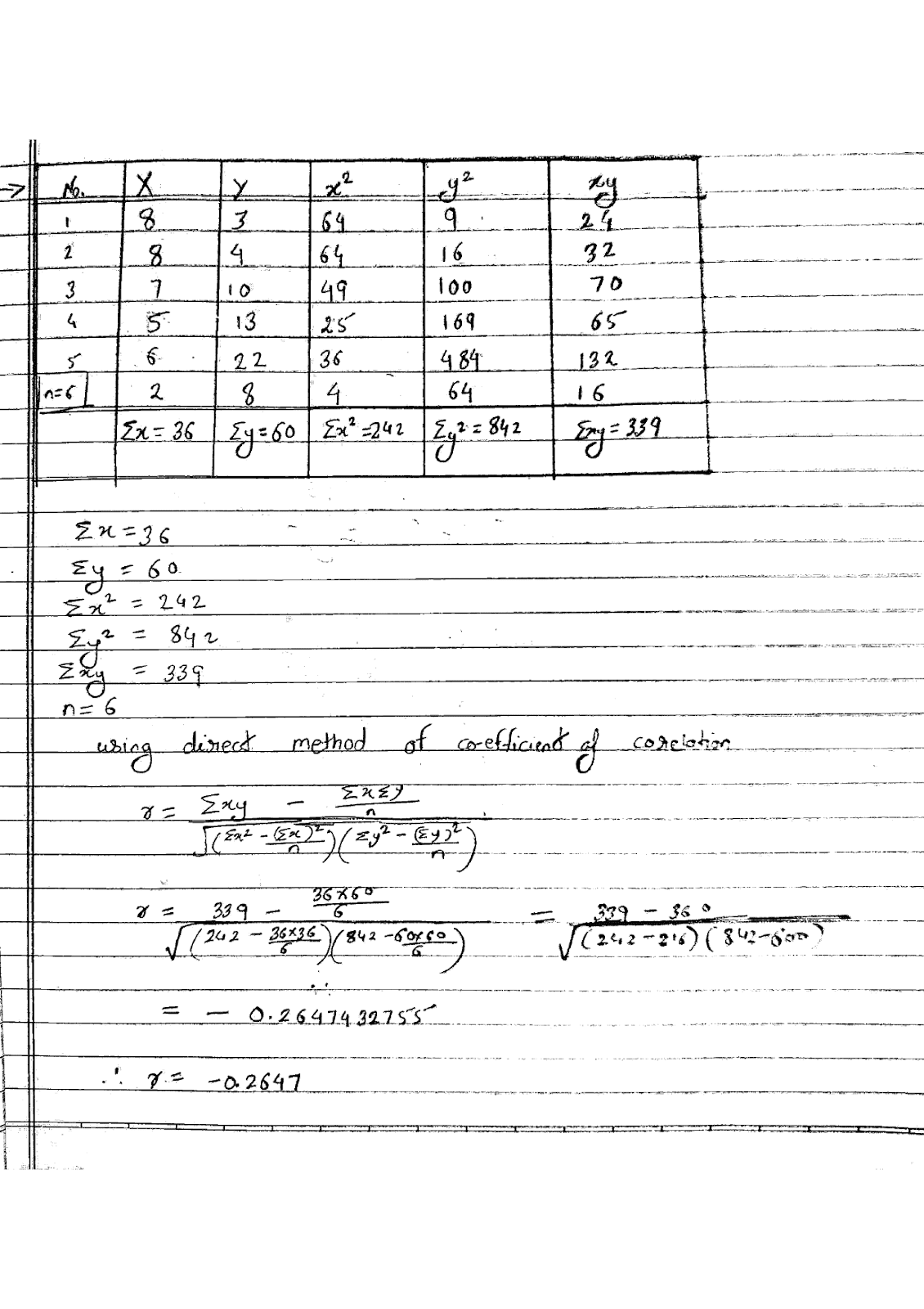# Calculate the coefficient of correlation between X and Y by direct method

more_vert

Calculate the coefficient of correlation between X and Y by direct method

X: 8,8,7,5,6,2
Y: 3,4,10,13,22,8

*Hint** Direct method formula is given by

$$r=\frac{\sum xy-\frac{\sum x\sum y}{n}}{\sqrt{(\sum x^2-\frac{(\sum x)^2}{n})(\sum y^2-\frac{(\sum y)^2}{n})}}$$

more_vert

verified
$$r=\frac{\sum xy-\frac{\sum x\sum y}{n}}{\sqrt{(\sum x^2-\frac{(\sum x)^2}{n})(\sum y^2-\frac{(\sum y)^2}{n})}}$$